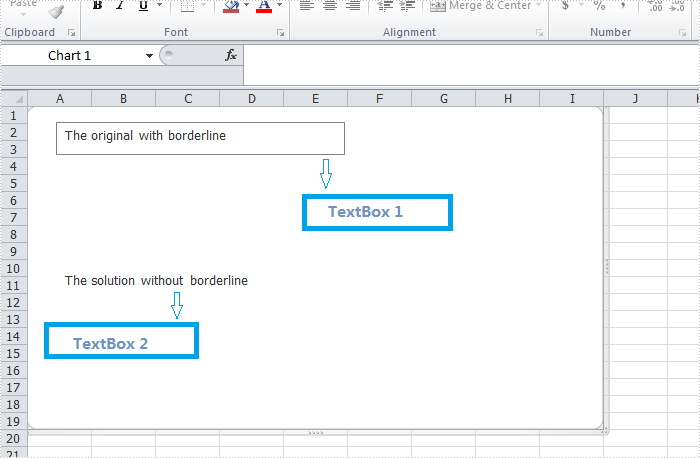Tuesday, 12 August 2014 07:57

## Remove borderline of textbox in Excel chart in C#, VB.NET

Written by  support iceblue
Rate this item

There is an article in the tutorials which demonstrates how to insert textbox with contents in Excel. Sometime back, a user of Spire.XLS wanted to know if it is possible to remove the borderline of the textbox that has been inserted in Excel chart. Yes, of course. This article focuses on delivering a solution to this issue.

In the following section, we're going to create two textboxes in the same chart, one textbox is built with borderline, the other one without. Then we can learn how to remove borderline using Spire.XLS by comparison.

#### Code snippet for remove borderline of textbox:

Step 1: Create a new instance of workbook.

```Workbook workbook = new Workbook();
workbook.Version=ExcelVersion.Version2010;
```

Step 2: Create a new worksheet named "Remove Borderline" and add a chart to the worksheet.

```Worksheet sheet = workbook.Worksheets;
sheet.Name = "Remove Borderline";
```

Step 3: Create textbox1 in the chart and input text information.

```chart.TextBoxes.AddTextBox(50, 50, 100, 500).ShapeType = ExcelShapeType.TextBox;
chart.TextBoxes.Text = "The original with borderline";
```

Step 4: Create textbox2 in the chart, input text information and remove borderline.

```XlsTextBoxShape textbox = chart.TextBoxes.AddTextBox(500, 50, 100, 500) as XlsTextBoxShape;
textbox.Text = "The solution without borderline";
textbox.Line.Weight = 0;
```

Step 5: Save and launch the file.

```workbook.SaveToFile("Sample.xlsx", ExcelVersion.Version2010);
Process.Start("Sample.xlsx");
```

Result:Full code:

[C#]
```Workbook workbook = new Workbook();
workbook.Version=ExcelVersion.Version2010;
Worksheet sheet = workbook.Worksheets;
sheet.Name = "Remove Borderline";
//original
chart.TextBoxes.AddTextBox(50, 50, 100, 500).ShapeType = ExcelShapeType.TextBox;
chart.TextBoxes.Text = "The original with borderline";
//solution
XlsTextBoxShape textbox = chart.TextBoxes.AddTextBox(500, 50, 100, 500) as XlsTextBoxShape;
textbox.Text = "The solution without borderline";
//set 0 then invisible
textbox.Line.Weight = 0;
workbook.SaveToFile("Sample.xlsx", ExcelVersion.Version2010);
Process.Start("Sample.xlsx");
```
[VB.NET]
```Dim workbook As New Workbook()
workbook.Version = ExcelVersion.Version2010
Dim sheet As Worksheet = workbook.Worksheets(0)
sheet.Name = "Remove Borderline"
Dim chart As Chart = sheet.Charts.Add()
'original
chart.TextBoxes.AddTextBox(50, 50, 100, 500).ShapeType = ExcelShapeType.TextBox
chart.TextBoxes(0).Text = "The original with borderline"
'solution
Dim textbox As XlsTextBoxShape = TryCast(chart.TextBoxes.AddTextBox(500, 50, 100, 500), XlsTextBoxShape)
textbox.Text = "The solution without borderline"
'set 0 then invisible
textbox.Line.Weight = 0
workbook.SaveToFile("Sample.xlsx", ExcelVersion.Version2010)
Process.Start("Sample.xlsx")
```